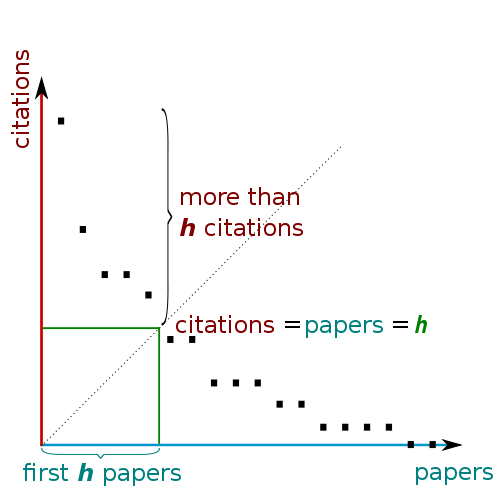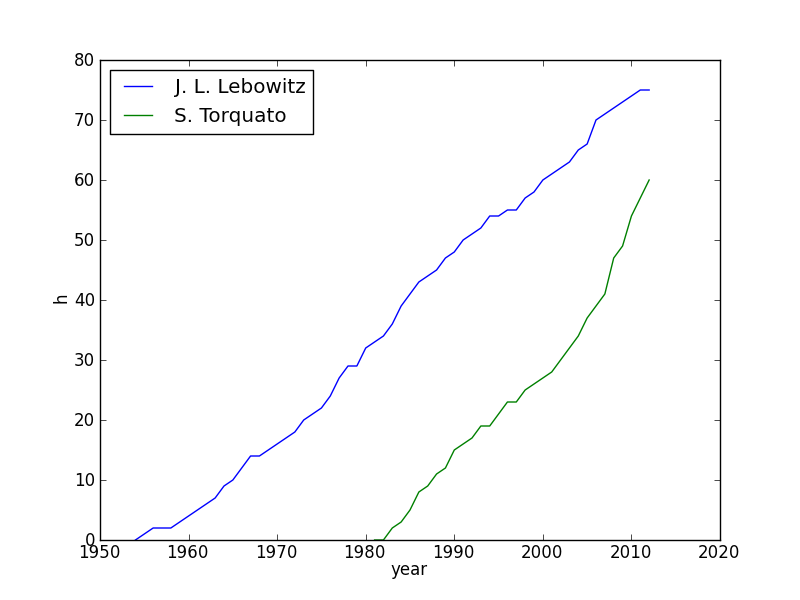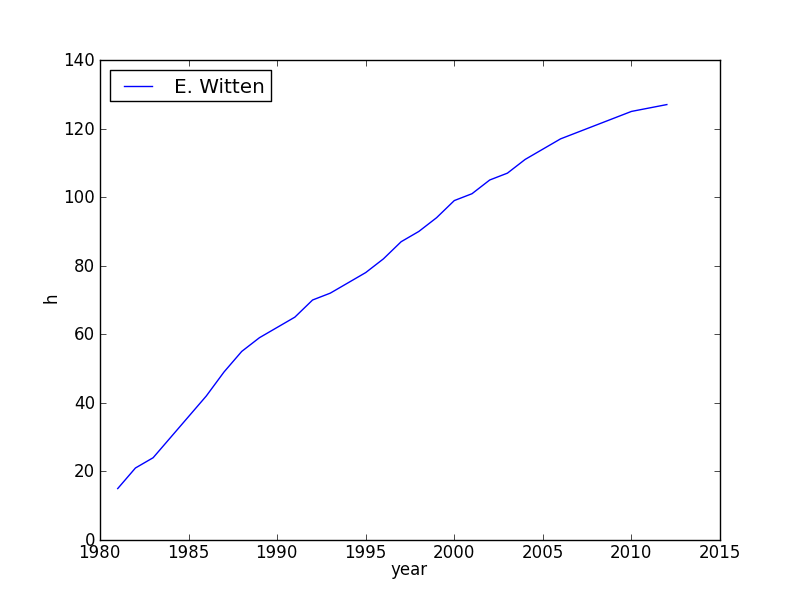# h-index

A long-standing question in academia has been how to quantify research productivity and impact. Measures such as the total number of publications or the total number of citations have obvious shortcomings. The field of bibliometry was revolutionized in 2005 when Jorge Hirsch proposed the h-index that provides a simultaneous measure of productivity and impact.

A scholar has index h if h of his or her N papers have at least h citations each, and the other (N - h) papers have at most h citations each. In order to calculate the h-index one first creates a rank-ordered list of all the publications of an author. Thus the first paper in the list has the most citations and the last the least (often zero). One then descends the list until the number of citations of an article is equal to its rank.Databases such as ISI Web of Science and Google Scholar provide automated calculation of the current h-index, but they do not compute its time evolution. The index can never decrease and may have a convex or concave shape or any combination.

Here are some examples of the evolution of the h-index for some prominent scientists:and another:Here is the Python program used to calculate the time evolution. To use the program follow the instructions. An sample input file can be found here.